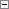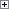To make it easy to maintain an application, you should follow a few strict guidelines when you write C/AL code. This section describes the general rules for:

• Spacing and indentation
• Parentheses
• Symbolic values
• Parameters
• Order in expressions
• Order of variables

In addition, we recommend that you enter all C/AL code as English (United States). If all code is in the same language, then it is easier to maintain the application.

Spacing and Indentation

The following examples show how to use spacing and indentation.

Example

Use exactly one space character on each side of a binary operator, such as assignment or plus.Copy Code
y := (a + b) / 100;

Example

Do not have a space between a unary operator and its argument.Copy Code
y := -x;

Example

Refer to multiple dimensions in a record array by using sets of brackets without space characters between the dimensions.Copy Code
a[i][j] := b;

Example

Do not use blank lines at the beginning or end of any functions.Copy Code
PROCEDURE P();
BEGIN
x := a;
y := (a + b) / 100;
END;

Example

In general, use an indentation of two character spaces.Copy Code
IF a <> '' THEN
Record.TESTFIELD(b);

Example

When you split a C/AL statement into two or more lines, do not align the continuation lines according to user-defined or system-defined variables, functions, field names, object names, and other elements. Instead, indent the continuation lines by two characters. Aligning by variable, function, field name, or object name may look clearer in your program, but the alignment will not hold if the variable name is changed to something shorter or longer. Although the system-defined variable and function names are not likely to change, the rule also applies when you use them.Copy Code
MyVariable :=
Variable1 + Variable2 * 2 +
Variable3 * 3;

ExampleCopy Code
MyFunction(
Expression1,Expression2,
Expression3,Expression4);

ExampleCopy Code
ERROR(
StringExpression,
Expression1,Expression2,Expression3);

ExampleCopy Code
IF NOT
SETCURRENTKEY(
aaaaaaaaaa,bbbbbbbbbb,cccccccccc,
dddddddddd,eeeeeeeeee)
THEN
SETCURRENTKEY(bbbbbbbbbb,aaaaaaaaaa);

Parentheses

The following examples show how to use parentheses.

Example

Align a opening parenthesis in an expression with a corresponding parenthesis on the preceding line.Copy Code
aaaaaaaaaa :=
((xxxxxxxxxxx / yyyyyyyyyyyyy) -
(1 + zzzzzzzzzz / 100)) *
100;

Example

Do not use parentheses in a function call if the function does not have any parameters.Copy Code
PostLine;

Use parentheses only to enclose compound expressions inside compound expressions.Copy Code
IF Boolean1 AND Boolean2 AND (x = y) THEN
x := a + b
ELSE
x := b / (a + b);

The following examples show how to format comments.

Example

Always start comments with // and one space character. Never use braces ({ and }). To emphasize a comment, put it on a separate line and insert one empty line before it.Copy Code
x := x + 1;
// Comment
x := x * 2;

Example

If the comment is on the same line as the C/AL code, then add one space character before the comment sign.Copy Code
x := '....'; // Comment

Symbolic Values

When possible, use the name of the Option for a field instead of just an integer for the value.

ExampleCopy Code
Table.Field := Table.Field::Option;

ExampleCopy Code
IF Table.Field IN[Table.Field::Option1,Table.Field::Option2] THEN
EXIT;

Incorrect ExampleCopy Code
Table.Field := 1;

Example

When possible, use the name of the Option in the conditional possibilities in a CASE statement.Copy Code
CASE xxx OF
xxx::aaa,xxx::bbb:
x := y;
ELSE
y := x;
END;

Parameters

Use parameters when you want to transfer information to a function.

To use a parameter as an option field, define it in the function. When you call the function, use an integer as parameter in the call.

ExampleCopy Code
...
P(0);
...
PROCEDURE P(MyOption : 'Value0,Value1,Value2');
BEGIN
CASE MyOption OF
MyOption::Value0:
x := x * 10;
MyOption::Value1:
x := x * 15;
MyOption::Value2:
x := x * 20;
END;
END;

Order in Expressions

The variable that you are operating on or comparing to something else must always come first in expressions.

IF x <> 0 THEN

x := x - 100;

Example

IF (Date < a) OR (Date > b) THEN

Date := c;

IF 0 > x THEN

x := x - 100;

Order of Variables

List variables in the following order:

• Record variables
• Page variables
• Report variables
• XMLport variables
• Codeunit variables
• Dialog, file, and BLOB variables
• Simple variables

Record variables are listed in an order that reflects the hierarchy of the tables that are used in the database. Base tables come before journals and other nonposted lines and headers, which themselves come before ledger entries and posted lines and headers.

ExampleCopy Code
VAR
GLSetup : Record 98;
UserSetup : Record 91;
ItemPostingGr : Record 94;
Item : Record 27;
ItemJnlTemplate : Record 82;
ItemJnlBatch : Record 233;
ItemJnlLine : Record 83;
ItemReg : Record 46;
ItemLedgEntry : Record 32;